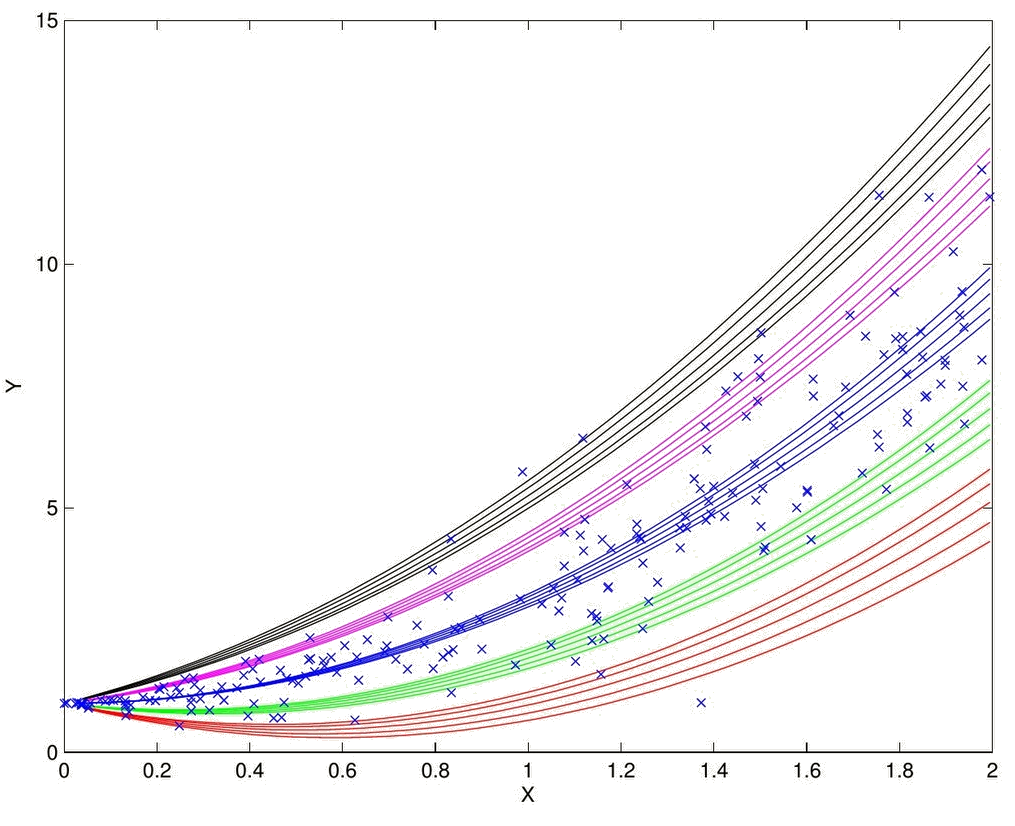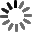El sitio web de la UCLM utiliza cookies propias y de terceros con fines técnicos y de análisis, pero no recaba ni cede datos de carácter personal de los usuarios. Sin embargo, puede haber enlaces a sitios web de terceros, con políticas de cookies distintas a la de la UCLM, que usted podrá aceptar o no cuando acceda a ellos.

Puede obtener más información en la Política de cookies. Aceptar# Bayesian methods OpenBUGS

Curso de fuentes OpenUCLM

This course is dedicated to describe in detail what Bayesian methods are, including prior, posterior and predictive distributions, and the use of Markov Chain Monte Carlo (MCMC) methods using OpenBUGS. We start with, a short description, with some examples, including the concept of conjugate distributions. We explain how these methods permit to convert a deterministic model into a random model by converting its parameters into random variables, as Bayesian method do, or to improve already random models into more complex ones using the same conversion. The OpenBUGS software is described and used to solve several classical methods in Statistics and Probability. We explain how the use of scripts can facilitate and speed up the simulation process. Apart form these examples, we include some fatigue models examples that show how we can even obtain the percentiles of percentile curves. This permit treating the randomness due to parameters.

Autores Fecha Enrique Castillo 17/09/2019 Ingles

Me GustaThe course is motivated and emphasis is made on using MCMC methods and OpenBUGS to transform deterministic models into random ones or performing a Bayesian analysis of already random models. With this tool, answers to problems can be given as density functions instead of simple point estimates or confidence intervals.

Presentation of the course

Enrique Castillo

This video is a summary of a course on OpenBUGS. The course starts with a motivation and a summary of Bayesian methods including the most important concepts, such as the basic moel, prior and posterior distributions, conjugate families, samples, and prior and posterior predictive distributions, which are illustrated with some examples. Next we explain how to use the Doodle tool to build the Bayesian network of the Bayesian model, which includes the model variables and also the model parameters. Next some examples of applications are discussed, such as the BPR travel time model, the parabolic regression model, the rate and the Pearson correlation model, etc. It is breafly explained how to work with scripts and use JAGS and matlab. Complete examples with the corresponding matlab codes are given for the readers to benefit from them and use them as starting work material to solve their problems.

OpenBUGS course motivation

Enrique Castillo

In this lesson we motivate Bayesian methods and mainly the OpenBUGS software. We show how we can convert a deterministic parameteric model into a random model by transforming its parameters into random variables. We also see how We can deal with Bayesian methods for random models by converting their parameters into random variables too. The OpenBUGS software makes this possible in a very simple manner.

In this block we introduce Bayesian methods, first, by giving the definitions of its main elements, and second, by means of some simple but illustrative examples. The concept of prior and posterior distributions are introduced and the formula to obtain the posterior, in terms of the prior and the sample likelihood is given. Prior and posterior predictive distributions are also defined and obtained. Some graphical examples are used to illustrate the new and deep concepts. The Doodle tool, to build the Bayesian network defining the models and their parameters is described in detail and some examples are given. Finally, an initial view of OpenBUGS is given using the BPR traffic model, which is converted from deterministic to random.

OpenBUGS Bayesian Methods

Enrique Castillo

The OpenBUGs course is introduced with a motivation based on its capabilities to convert deterministic models into random models and to analyze already random models from a Bayesian point of view. We also introduce what Bayesian methods are, including prior, posterior and predictive distributions..

The Doodle tool in OpenBUGS

Enrique Castillo

We describe how to build the acyclic graph of the Bayesian network that describes the existing dependencies among the variables and the parameters of the model. From this graph we can generate automatically the commands to be given to OpenBUGS. A complete and detailed example is given to illustrate all the steps of the model construction process.

An initial view of OpenBUGS

Enrique Castillo

A simple example is described, in which all necessary steps to run OpenBUGS are explained in detail. Before trying to use the software, it is very convenient to take a look to this lesson. The BPR function is used as an illustrative example.

How to use OpenBUGS

Enrique Castillo

This video explains, step by step, how to use OpenBUGS using an example. A detailed explanation is given of how to provide OpenBUGS the model commands, the data and the initial files. The video ends with an explanation of how to analyze the results and get some plots of the main results.

Calling OpenBUGS from matlab

Enrique Castillo

This video shows how to call OpenBUGS from matlsab. We use the BPR model example and describe in detail how to write the commands, the data and the initial files from matlab and how to call OpenGUBS and JAGS from matlab. Finally, we indicate how to recover the simulated data from OpenBUGS and how to plot some interesting plots, such as the history plots of the simulated parameter values and the corresponding densities.

We dedicate this second block to some statistical applications. We start with the problem of estimating a rate considering two cases: (a) the sample size is known, and (b) it is unknown. As already known, if the parameters are assumed to be random we get a Bayesian method to analyze this problem. Some interesting dependencies of the parameters will be discovered. The second example is the Pearson correlation example, in which this statistic is considered as a random variable, instead of a deterministic value. This allows us to answer the problem of finding the Pearson correlation of a model by means of a density function instead of a point or confidence estimate, which goes further beyond than the classical statistics.

We describe an application consisting in estimating a rate or proportion in two different cases: (1) when we know the sample size, and (2) when the sample size is unknown. We show how the problem of estimating a proportion can be solved using Bayesian methods based on Markov Chain Monte Carlo methods.
We describe how to estimate the Pearson correlation coefficient using a Bayesian method Markov Chain Monte Carlo method. The final answer is neither a point estimate ,nor a confidence interval, but a density estimate. To solve the problem, the OpenBUGS software is used.

This block is dedicated to some programming tools that facilitate the use of Bayesian MCMC methods. We start with scripts, that allow us to use the software without the mouse, i. e., using program commands called scripts. In this way the speed in getting the results increases substantially. Next, we describe the JAGS tool, an alternative to the standard OpenBUGS, that allows as to compare results with a small extra work. The call to JAGS from Matlab is described. Finally, we explain how to use OpenBUGS from Matlab, by explaining how to generate the commands, the data and the initial files and how to generate scripts automatically and call then OpenBUGS later to initiate the simulation process.

Scripts in OpenBUGS

Enrique Castillo

In this lecture we describe how tje OpenBUGS software can be used by means of scripts. The scripts are series of commands that permit executing the software very rapidly. Another advantage is that we can copy and paste the scripts and, making very small changes, we can adapt them for other example or application.

How to use OpenBUGS scripts

Enrique Castillo

This video describes how to run OpenBUGS using scripts and illustrates how we can save a lot of time avoiding menus, buttons, mouse and the keyboard.

OpenBUGS with JAGS

Enrique Castillo

We describe how the matlab software can be used to solve problems uding JAGS. Some examples of applications are sed to illustrate the computer programs. The matlab programs for some of the applications described in the course are listed at the end of the course.

OpenBUGS in Matlab

Enrique Castillo

We describe how the matlab software can be used to solve problems by OpenBUGS. Some examples of applications are used to illustrate the computer programs. The matlab programs for some of the applications described in the course are listed at the end of the course.

In this part we describe some engineering applications. We start with a heteroscedastic parabolic regression model, in which the parameters are considered as random instead of deterministic. Since in the deterministic version we can plot percentile regression curves, with this model each percentile curve has its own percentiles curves. The second example is a powerful non-linear random fatigue example, which is selected to satisfy some engineering, physical and statistical conditions and is enriched by assuming that its five parameters are random variables. In this way, each of the S-N percentile curves of the model can be given as stochastic processes, whose percentiles are obtained. Several deal data sets are used to fit the models.

We describe how to estimate an heteroscedastic parabolic regression model using a Bayesian method Markov Chain Monte Carlo method. To solve the problem, the OpenBUGS software is used. An interesting novelty is that we can give densities instead of point or confidence interval estimates, and the percentiles curves are estimated by confidence bands.

Fatigue models with OpenBUGS

Enrique Castillo

The stochastic Castillo-Fernández Canteli fatigue model for the S-N curves is described. The parameters of the randm model are assumed to be random and then, we have a Bayesian model. Real data from Maennig and Holmen are used to illustrate the good resulting fit of the model. In particular, the percentiles of the percentiles curves are obtained as an original result.

This block is dedicated to provide the readers with some of the above examples implemented in matlab and/or JAGS. The whole listing of the programs are given, so that they can immediately run the programs and used them as templates to write their own examples. The complete results, figures, tables and files are automatically saved in a folder, whose name is selected by the user. I hope they enjoy with this experience that can change the way they work with deterministic and statistical models in the furure. If this is so, I will be happy of the change and progress this attitude and orientation implies.

The BPR Example

Enrique Castillo

The Rate Model Example

Enrique Castillo

The Fatigue Examples

Enrique Castillo

Here are all the slideshows for Bayesian methods OpenBUGS

Slideshows

Enrique Castillo

Here are all the slideshows for Bayesians methods OpenBUGS